# DNN text-to-speech synthesis (jp)¶

Source code: https://github.com/r9y9/nnmnkwii_gallery

DNN日本語音声合成のデモです。基本的な処理は言語非依存なので、DNN英語音声合成の場合とほとんど変わりません。変更点をまとめると、

• CMU ARCTICの代わりに、HTS-demo-NIT-ATR503 のデータを使います。ライセンス
• State-levelでアライメントされたコンテキストラベルではなく、phone-levelでアライメントされたものを使います。
• Phone-levelのアライメントを使う都合上、言語特徴量で使えるframe-level featureが変わります（少なくなります）。具体的には、subphone_features="full" ではなく、 subphone_features="corase_coding" として、言語特徴量を抽出します。subphone_featuresとは何なのか、といった説明は現状のドキュメントにはないので、詳しく知りたい方は Merlinのソースコード をご覧ください。
• 言語特徴量の周出に質問ファイルは、HTSのコンテキストクラスタリングで使われるファイルを元に、少し修正したものを使っています。

このデモノートブックを実行するためには、以下示すpythonパッケージが必要なのに加えて、HTS-demo_NIT-ATR503-M001 をダウンロードし、任意の場所に展開しておく必要があります。HTSをインストールする必要はありません。

This notebook demonstrates how to build DNN-based speech synthesis system. We will investigate very simple feed forward neural networks. Steps are composed of:

• Linguistic/Duration/Acoustic feature extraction
• Dataset preparation
• Training duration and acoustic models
• Speech parameter generation and waveform synthesis

Generated audio examples are attached at the bottom of the notebook. For simplicity, feature extraction steps will be performed with an external python script (200 lines). To run the notebook, in addition to nnmnkwii and its dependencies, you will need the following packages:

Please make sure that you have all dependneices if you are trying to run the notebook locally.

pip install pysptk pyworld librosa tqdm docopt

Part of code is adapted from Merlin. Speech analysis/synthesis is done by pysptk and pyworld. Librosa is used to visualize features. PyTorch is used to build DNN models.

The notebook requires wav files with aligned HTS-style full-context lablel files. You can download the necessary files by the following script.

In :
from os.path import expanduser, join
# 以下を、ダウンロードしたデモのディレクリを指すように、修正してください。
HTS_DEMO_ROOT = join(expanduser("~"), "local", "HTS-demo_NIT-ATR503-M001")

In :
! ./scripts/copy_from_htsdemo.sh $HTS_DEMO_ROOT  HTS-demo_NIT-ATR503-M001 already copied  In : %pylab inline rcParams["figure.figsize"] = (16,5) from nnmnkwii.datasets import FileDataSource, FileSourceDataset from nnmnkwii.datasets import MemoryCacheFramewiseDataset from nnmnkwii.util import trim_zeros_frames, remove_zeros_frames from nnmnkwii.util import minmax, meanvar, minmax_scale, scale from nnmnkwii import functions as F from nnmnkwii.io import hts from nnmnkwii.frontend import merlin as fe from nnmnkwii.postfilters import merlin_post_filter from os.path import join, expanduser, basename, splitext, basename, exists import os from glob import glob import numpy as np from scipy.io import wavfile from sklearn.model_selection import train_test_split import pyworld import pysptk import librosa import librosa.display import IPython from IPython.display import Audio  Populating the interactive namespace from numpy and matplotlib  ## Data¶ In this demo we construct datasets from pre-computed linguistic/duration/acoustic features because computing features from wav/label files on-demand are peformance heavy, particulary for acoustic features. See the following python script if you are interested in how we extract features. In : DATA_ROOT = "./data/NIT-ATR503/" test_size = 0.01 # This means 480 utterances for training data random_state = 1234  In : ! python ./scripts/prepare_features.py$DATA_ROOT --use_phone_alignment --question_path="./data/questions_jp.hed"

Features for duration model training found, skipping feature extraction.
Features for acousic model training found, skipping feature extraction.


### Data specification¶

Almost same as Merlin's slt_arctic demo. The only difference is that frequency warping paramter alpha is set to 0.41, instead of 0.58. As far as I know 0.41 is the best parameter approximating mel-frequency axis for 16kHz-sampled audio signals.

In :
mgc_dim = 180
lf0_dim = 3
vuv_dim = 1
bap_dim = 15

duration_linguistic_dim = 1119
acoustic_linguisic_dim = 1123
duration_dim = 1
acoustic_dim = mgc_dim + lf0_dim + vuv_dim + bap_dim

import pysptk
import pyworld

fs = 48000
frame_period = 5
fftlen = pyworld.get_cheaptrick_fft_size(fs)
alpha = pysptk.util.mcepalpha(fs)
hop_length = int(0.001 * frame_period * fs)

mgc_start_idx = 0
lf0_start_idx = 180
vuv_start_idx = 183
bap_start_idx = 184

windows = [
(0, 0, np.array([1.0])),
(1, 1, np.array([-0.5, 0.0, 0.5])),
(1, 1, np.array([1.0, -2.0, 1.0])),
]


### File data sources¶

We need to specify 1) where to find pre-computed features and 2) how to process them. In this case,

1. collect_files : Collects .bin files. External python script writes files in binary format. Also we split the files into train/test set.
2. collect_features : Just load from file by np.fromfile.
In :
class BinaryFileSource(FileDataSource):
def __init__(self, data_root, dim, train):
self.data_root = data_root
self.dim = dim
self.train = train
def collect_files(self):
files = sorted(glob(join(self.data_root, "*.bin")))
train_files, test_files = train_test_split(files, test_size=test_size,
random_state=random_state)
if self.train:
return train_files
else:
return test_files
def collect_features(self, path):
return np.fromfile(path, dtype=np.float32).reshape(-1, self.dim)


Then we can construct datasets for duraton and acoustic models. We wil have

• X: Input (duration, acoustic) datasets
• Y: Target (duration, acoustic) datasets

Note that dataset itself doesn't keep features in memory. It loads features on-demand while iteration or indexing.

In :
X = {"duration":{}, "acoustic": {}}
Y = {"duration":{}, "acoustic": {}}
utt_lengths = {"duration":{}, "acoustic": {}}
for ty in ["duration", "acoustic"]:
for phase in ["train", "test"]:
train = phase == "train"
x_dim = duration_linguistic_dim if ty == "duration" else acoustic_linguisic_dim
y_dim = duration_dim if ty == "duration" else acoustic_dim
X[ty][phase] = FileSourceDataset(BinaryFileSource(join(DATA_ROOT, "X_{}".format(ty)),
dim=x_dim,
train=train))
Y[ty][phase] = FileSourceDataset(BinaryFileSource(join(DATA_ROOT, "Y_{}".format(ty)),
dim=y_dim,
train=train))
# this triggers file loads, but can be neglectable in terms of performance.
utt_lengths[ty][phase] = [len(x) for x in X[ty][phase]]


### Utterance lengths¶

Let's see utterance lengths histrogram.

In :
print("Total number of utterances:", len(utt_lengths["duration"]["train"]))
print("Total number of frames:", np.sum(utt_lengths["duration"]["train"]))
hist(utt_lengths["duration"]["train"], bins=64);

Total number of utterances: 497
Total number of frames: 28435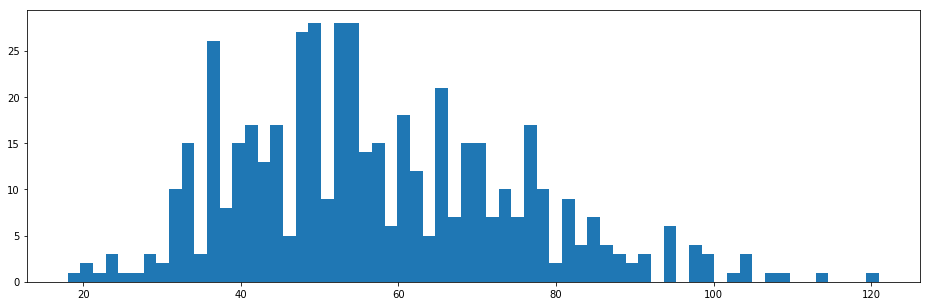In :
print("Total number of utterances:", len(utt_lengths["acoustic"]["train"]))
print("Total number of frames:", np.sum(utt_lengths["acoustic"]["train"]))
hist(utt_lengths["acoustic"]["train"], bins=64);

Total number of utterances: 497
Total number of frames: 483363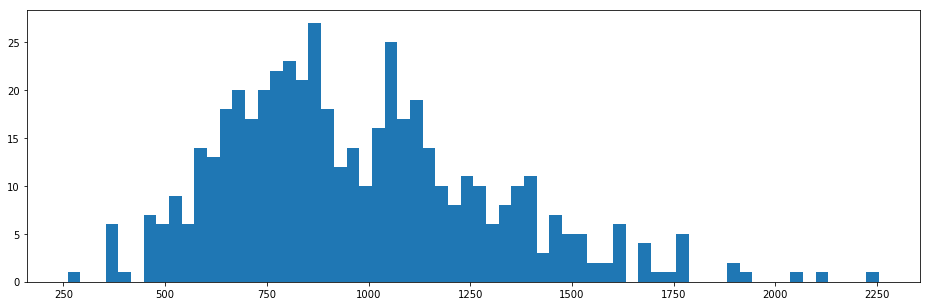### How data look like?¶

Pick an utterance from training data and visualize its features.

In :
def vis_utterance(X, Y, lengths, idx):
"""Visualize the following features:

1. Linguistic features
2. Spectrogram
3. F0
4. Aperiodicity
"""
x = X[idx][:lengths[idx]]
y = Y[idx][:lengths[idx]]

figure(figsize=(16,20))
subplot(4,1,1)
# haha, better than text?
librosa.display.specshow(x.T, sr=fs, hop_length=hop_length, x_axis="time", cmap="magma")

subplot(4,1,2)
logsp = np.log(pysptk.mc2sp(y[:,mgc_start_idx:mgc_dim//len(windows)], alpha=alpha, fftlen=fftlen))
librosa.display.specshow(logsp.T, sr=fs, hop_length=hop_length, x_axis="time", y_axis="linear", cmap="magma")

subplot(4,1,3)
lf0 = y[:,mgc_start_idx]
vuv = y[:,vuv_start_idx]
plot(lf0, linewidth=2, label="Continuous log-f0")
plot(vuv, linewidth=2, label="Voiced/unvoiced flag")
legend(prop={"size": 14}, loc="upper right")

subplot(4,1,4)
bap = y[:,bap_start_idx:bap_start_idx+bap_dim//len(windows)]
bap = np.ascontiguousarray(bap).astype(np.float64)
aperiodicity = pyworld.decode_aperiodicity(bap, fs, fftlen)
librosa.display.specshow(aperiodicity.T, sr=fs, hop_length=hop_length, x_axis="time", y_axis="linear", cmap="magma")
# colorbar()

In :
idx = 0 # any
vis_utterance(X["acoustic"]["train"], Y["acoustic"]["train"], utt_lengths["acoustic"]["train"], idx)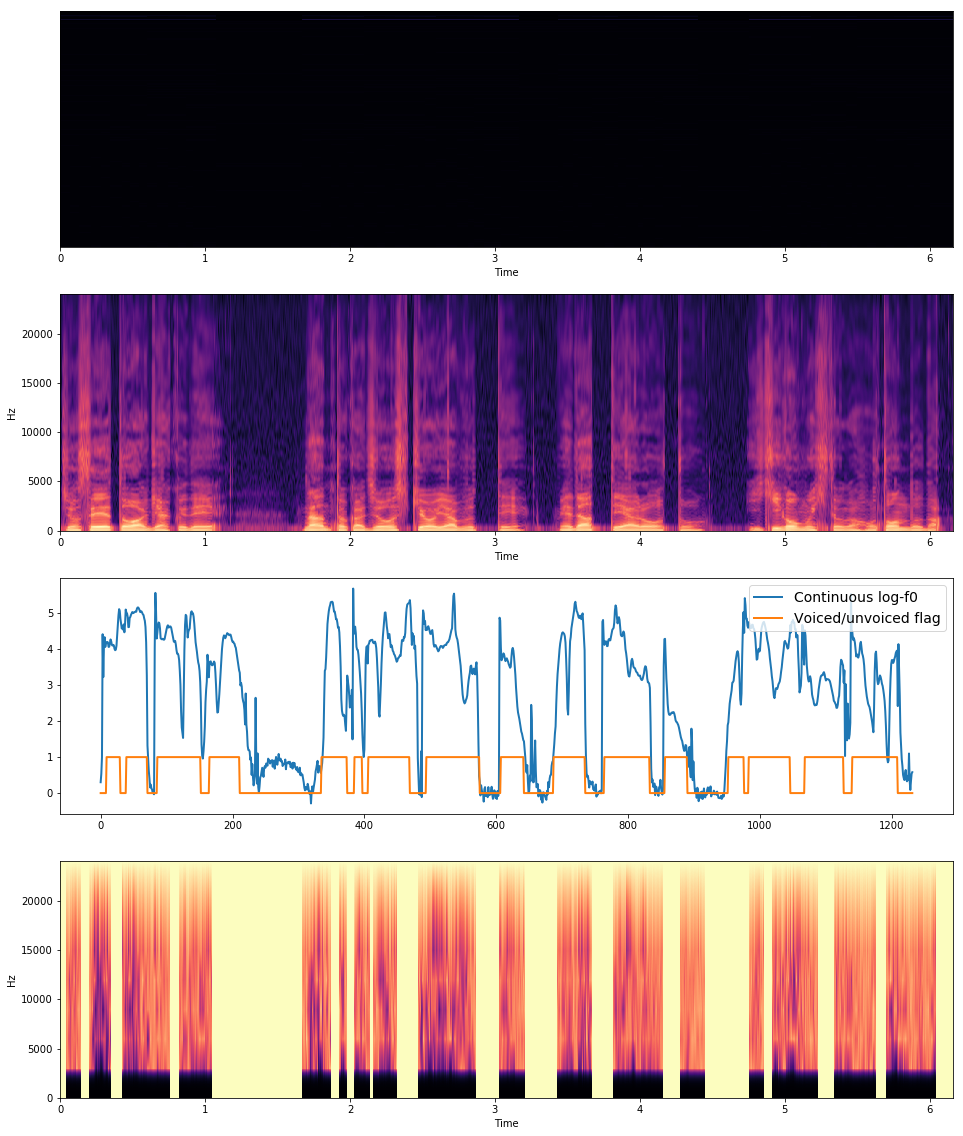As you can see the top of the images, linguistic features are not clear. This is because linguistic features have very differnt scale for each dimention. This will be clear after normalization.

### Statistics¶

Before training neural networks, we need to normalize data. Following Merlin's demo script, we will apply min/max normalization for linguistic features and mean/variance normalization to duration/acoustic features. You can compute necessary statistics using nnmnkwii.util.minmax and nnmnkwii.util.meanvar. The comptuation is online, so we can use the functionality for any large dataset.

In :
X_min = {}
X_max = {}
Y_mean = {}
Y_var = {}
Y_scale = {}

for typ in ["acoustic", "duration"]:
X_min[typ], X_max[typ] = minmax(X[typ]["train"], utt_lengths[typ]["train"])
Y_mean[typ], Y_var[typ] = meanvar(Y[typ]["train"], utt_lengths[typ]["train"])
Y_scale[typ] = np.sqrt(Y_var[typ])


Linguistic features should be clear with normalization as shown below.

In :
idx = 0
typ = "acoustic"
x = X[typ]["train"][idx][:utt_lengths[typ]["train"][idx]]
x = minmax_scale(x, X_min[typ], X_max[typ], feature_range=(0.01, 0.99))
librosa.display.specshow(x.T, sr=fs, hop_length=hop_length, x_axis="time")
colorbar()

Out:
<matplotlib.colorbar.Colorbar at 0x7f0ba1c3ba90>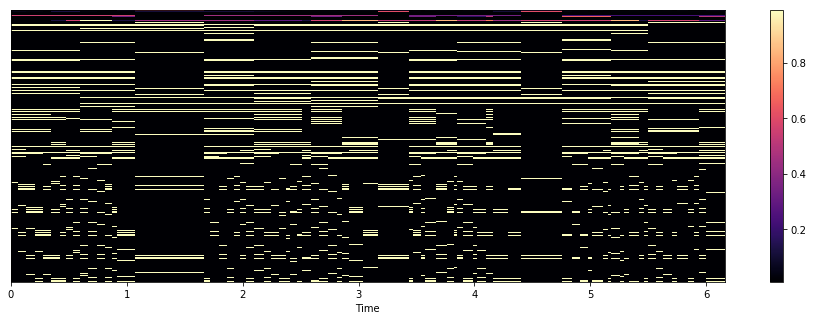### Combine datasets and normalization.¶

In this demo we use PyTorch to build DNNs. PyTorchDataset is just a glue Dataset wrapper, which combines our dataset and normalization.

In :
from torch.utils import data as data_utils
import torch

class PyTorchDataset(torch.utils.data.Dataset):
"""Thin dataset wrapper for pytorch

This does just two things:
1. On-demand normalization
2. Returns torch.FlaotTensor instead of numpy.ndarray
"""
def __init__(self, X, Y, X_min, X_max, Y_mean, Y_scale):
self.X, self.Y = X, Y
self.X_min, self.X_max = X_min, X_max
self.Y_mean, self.Y_scale = Y_mean, Y_scale
def __getitem__(self, idx):
x, y = self.X[idx], self.Y[idx]
x = minmax_scale(x, self.X_min, self.X_max, feature_range=(0.01, 0.99))
y = scale(y, self.Y_mean, self.Y_scale)
x, y = torch.from_numpy(x), torch.from_numpy(y)
return x, y
def __len__(self):
return len(self.X)


## Model¶

We use pretty simple deep feed forward neural networks.

In :
import torch
from torch import nn
from tqdm import tnrange, tqdm
import torch
import torch.nn as nn
from torch import optim

In :
class MyNet(torch.nn.Module):
"""Very simple deep neural networks.
"""
def __init__(self, D_in, H, D_out, num_layers=2):
super(MyNet, self).__init__()
self.first_linear = nn.Linear(D_in, H)
self.hidden_layers = nn.ModuleList(
[nn.Linear(H, H) for _ in range(num_layers)])
self.last_linear = nn.Linear(H, D_out)
self.relu = nn.Tanh()

def forward(self, x):
h = self.relu(self.first_linear(x))
for hl in self.hidden_layers:
h = self.relu(hl(h))
return self.last_linear(h)


## Train¶

### Configurations¶

Network hyper parameters and training configurations (learning rate, weight decay, etc).

In :
num_hidden_layers = 3
hidden_size = 512
batch_size = 256
# We use PyTorch's multiprocess iterator. Note that large n_workers causes
# dataset copies across proccess.
n_workers = 2
pin_memory = True
nepoch = 20
lr = 0.001
weight_decay = 1e-6
use_cuda = torch.cuda.is_available()
print(use_cuda)

True


### Training loop¶

Our network predicts output features frame-by-frame, so we need to convert our datasets to support frame-wise iteration. MemoryCacheFramewiseDataset can do this with cache functionality. The training loop is pretty simple.

In :
def train(model, optimizer, X, Y, X_min, X_max, Y_mean, Y_scale,
utt_lengths, cache_size=1000):
if use_cuda:
model = model.cuda()

X_train, X_test = X["train"], X["test"]
Y_train, Y_test = Y["train"], Y["test"]
train_lengths, test_lengths = utt_lengths["train"], utt_lengths["test"]

X_train_cache_dataset = MemoryCacheFramewiseDataset(
X_train, train_lengths, cache_size)
Y_train_cache_dataset = MemoryCacheFramewiseDataset(
Y_train, train_lengths, cache_size)
train_dataset = PyTorchDataset(X_train_cache_dataset, Y_train_cache_dataset,
X_min, X_max, Y_mean, Y_scale)
train_dataset, batch_size=batch_size, num_workers=n_workers,
pin_memory=pin_memory, shuffle=True)
print("Train dataset number of frames", len(train_dataset))

X_test_cache_dataset = MemoryCacheFramewiseDataset(
X_test, test_lengths, cache_size)
Y_test_cache_dataset = MemoryCacheFramewiseDataset(
Y_test, test_lengths, cache_size)
test_dataset = PyTorchDataset(X_test_cache_dataset, Y_test_cache_dataset,
X_min, X_max, Y_mean, Y_scale)
test_dataset, batch_size=batch_size, num_workers=n_workers,
pin_memory=pin_memory, shuffle=False)
print("Test dataset numer of frames", len(test_dataset))

# Training loop
criterion = nn.MSELoss()
model.train()
print("Start frame-wise training...")
loss_history = {"train": [], "test": []}
for epoch in tnrange(nepoch):
for phase in ["train", "test"]:
if phase == "train":
model.train()
else:
model.eval()
running_loss = 0.0
if use_cuda:
x, y = x.cuda(), y.cuda()
x, y = Variable(x), Variable(y)
y_hat = model(x)
loss = criterion(y_hat, y)
if phase == "train":
loss.backward()
optimizer.step()
running_loss += loss.data

return loss_history


### Define models¶

In :
models = {}
for typ in ["duration", "acoustic"]:
models[typ] = MyNet(X[typ]["train"].shape[-1],
hidden_size, Y[typ]["train"].shape[-1],
num_hidden_layers)
print("Model for {}\n".format(typ), models[typ])

Model for duration
MyNet (
(first_linear): Linear (1119 -> 512)
(hidden_layers): ModuleList (
(0): Linear (512 -> 512)
(1): Linear (512 -> 512)
(2): Linear (512 -> 512)
)
(last_linear): Linear (512 -> 1)
(relu): Tanh ()
)
Model for acoustic
MyNet (
(first_linear): Linear (1123 -> 512)
(hidden_layers): ModuleList (
(0): Linear (512 -> 512)
(1): Linear (512 -> 512)
(2): Linear (512 -> 512)
)
(last_linear): Linear (512 -> 199)
(relu): Tanh ()
)


### Training Duration model¶

In :
ty = "duration"
loss_history = train(models[ty], optimizer, X[ty], Y[ty],
X_min[ty], X_max[ty], Y_mean[ty], Y_scale[ty], utt_lengths[ty])

Train dataset number of frames 28435
Test dataset numer of frames 317
Start frame-wise training...


In :
plot(loss_history["train"], linewidth=2, label="Train loss")
plot(loss_history["test"], linewidth=2, label="Test loss")
legend(prop={"size": 16})

Out:
<matplotlib.legend.Legend at 0x7f0b8c2e8f98>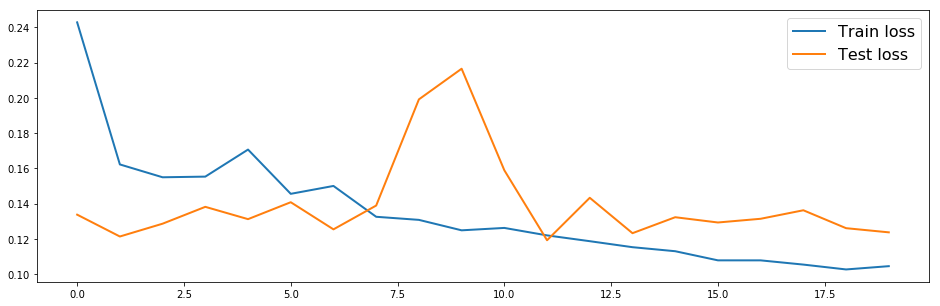### Training acoustic model¶

In :
ty = "acoustic"
loss_history = train(models[ty], optimizer, X[ty], Y[ty],
X_min[ty], X_max[ty], Y_mean[ty], Y_scale[ty], utt_lengths[ty])

Train dataset number of frames 483363
Test dataset numer of frames 5588
Start frame-wise training...


In :
plot(loss_history["train"], linewidth=2, label="Train loss")
plot(loss_history["test"], linewidth=2, label="Test loss")
legend(prop={"size": 16})

Out:
<matplotlib.legend.Legend at 0x7f0b8c226ef0>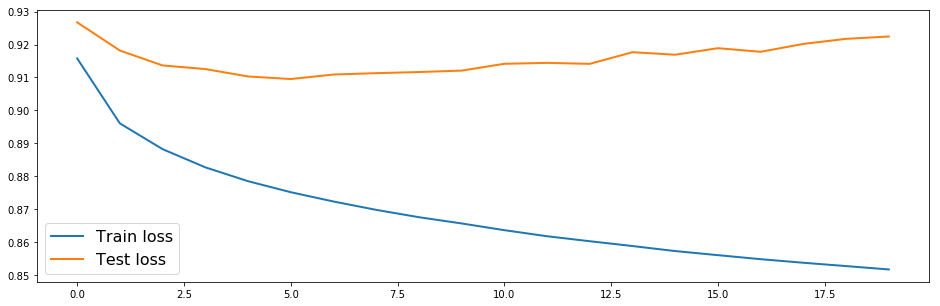## Test¶

Let's see how our network works.

### Parameter generation utilities¶

Not going to explain in details, but I think it's fairly simple and easy to understand. One thing I should note that is we are using nnmnkwii.postfilters.merlin_post_filter, which does same thing in Merlin's default post filter.

In :
binary_dict, continuous_dict = hts.load_question_set("./data/questions_jp.hed")

def gen_parameters(y_predicted):
# Number of time frames
T = y_predicted.shape

# Split acoustic features
mgc = y_predicted[:,:lf0_start_idx]
lf0 = y_predicted[:,lf0_start_idx:vuv_start_idx]
vuv = y_predicted[:,vuv_start_idx]
bap = y_predicted[:,bap_start_idx:]

# Perform MLPG
ty = "acoustic"
mgc_variances = np.tile(Y_var[ty][:lf0_start_idx], (T, 1))
mgc = F.mlpg(mgc, mgc_variances, windows)
lf0_variances = np.tile(Y_var[ty][lf0_start_idx:vuv_start_idx], (T,1))
lf0 = F.mlpg(lf0, lf0_variances, windows)
bap_variances = np.tile(Y_var[ty][bap_start_idx:], (T, 1))
bap = F.mlpg(bap, bap_variances, windows)

return mgc, lf0, vuv, bap

def gen_waveform(y_predicted, do_postfilter=False):
y_predicted = trim_zeros_frames(y_predicted)

# Generate parameters and split streams
mgc, lf0, vuv, bap = gen_parameters(y_predicted)

if do_postfilter:
mgc = merlin_post_filter(mgc, alpha)

spectrogram = pysptk.mc2sp(mgc, fftlen=fftlen, alpha=alpha)
aperiodicity = pyworld.decode_aperiodicity(bap, fs, fftlen)
f0 = lf0.copy()
f0[vuv < 0.5] = 0
f0[np.nonzero(f0)] = np.exp(f0[np.nonzero(f0)])

generated_waveform = pyworld.synthesize(f0.flatten().astype(np.float64),
spectrogram.astype(np.float64),
aperiodicity.astype(np.float64),
fs, frame_period)
return generated_waveform

def gen_duration(label_path, duration_model):
# Linguistic features for duration
duration_linguistic_features = fe.linguistic_features(hts_labels,
binary_dict, continuous_dict,
subphone_features=None).astype(np.float32)

# Apply normalization
ty = "duration"
duration_linguistic_features = minmax_scale(
duration_linguistic_features, X_min[ty], X_max[ty], feature_range=(0.01, 0.99))

# Apply model
duration_model = duration_model.cpu()
duration_model.eval()
x = Variable(torch.from_numpy(duration_linguistic_features)).float()
duration_predicted = duration_model(x).data.numpy()

# Apply denormalization
duration_predicted = duration_predicted * Y_scale[ty] + Y_mean[ty]
duration_predicted = np.round(duration_predicted)

# Set minimum state duration to 1
duration_predicted[duration_predicted <= 0] = 1
hts_labels.set_durations(duration_predicted)

return hts_labels

def test_one_utt(label_path, duration_model, acoustic_model, post_filter=True):
# Predict durations
duration_modified_hts_labels = gen_duration(label_path, duration_model)

# Linguistic features
linguistic_features = fe.linguistic_features(duration_modified_hts_labels,
binary_dict, continuous_dict,
subphone_features="coarse_coding")
# Trim silences
indices = duration_modified_hts_labels.silence_frame_indices()
linguistic_features = np.delete(linguistic_features, indices, axis=0)

# Apply normalization
ty = "acoustic"
linguistic_features = minmax_scale(linguistic_features,
X_min[ty], X_max[ty], feature_range=(0.01, 0.99))

# Predict acoustic features
acoustic_model = acoustic_model.cpu()
acoustic_model.eval()
x = Variable(torch.from_numpy(linguistic_features)).float()
acoustic_predicted = acoustic_model(x).data.numpy()

# Apply denormalization
acoustic_predicted = acoustic_predicted * Y_scale[ty] + Y_mean[ty]

return gen_waveform(acoustic_predicted, post_filter)


### Listen generated audio¶

Generated audio samples with Merlin's slt_full_demo are attached. You can compare them below.

In :
test_label_paths = sorted(glob(join(DATA_ROOT, "test_label_phone_align", "*.lab")))
hts_generated_wav_files = sorted(glob(join("./generated/hts_nit_atr503_2mix/*.wav")))

# Save generated wav files for later comparison
save_dir = join("./generated/jp-01-tts")
if not exists(save_dir):
os.makedirs(save_dir)

for label_path, wav_path in zip(test_label_paths, hts_generated_wav_files):
print("MyNet")
waveform = test_one_utt(label_path, models["duration"], models["acoustic"])
wavfile.write(join(save_dir, basename(wav_path)), rate=fs, data=waveform)
IPython.display.display(Audio(waveform, rate=fs))

print("HTS (NIT_ATR503 2mix, generated with default configurations)")

MyNet

HTS (NIT_ATR503 2mix, generated with default configurations)